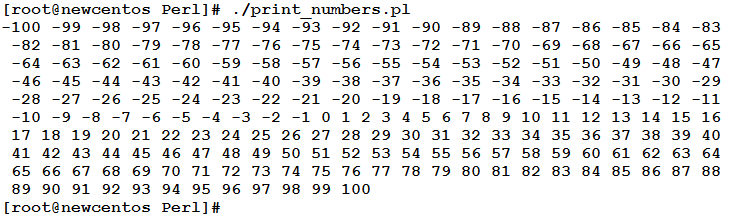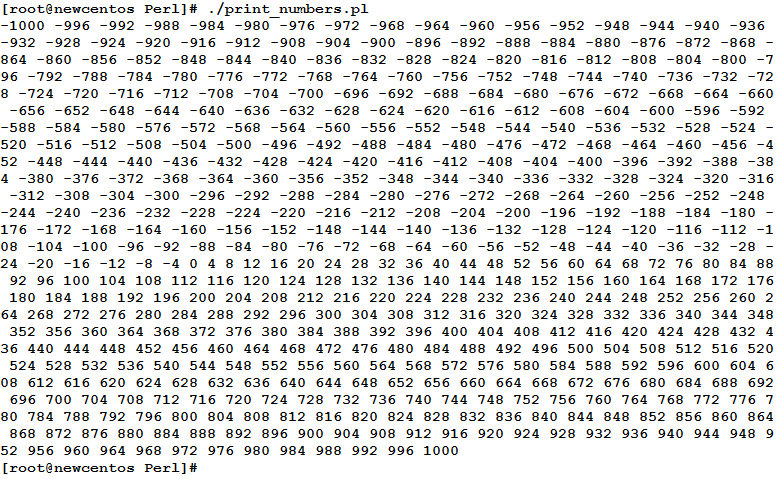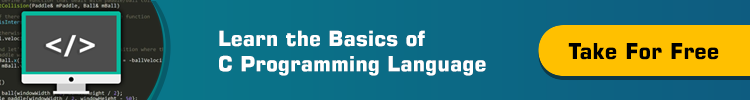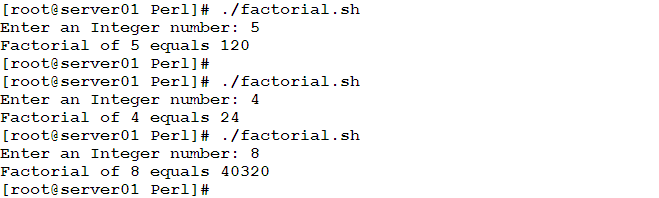# Learn about For Loop in Perl Programming

0
2272In computer programming, Loop refers to executing a block of code repeatedly. So, why should we learn about Loops? Your manager has asked you to write down numbers from 1 to 100, each in a separate line.
Okay, that is easy and manageable. What if he asked you to write from 1 to 1000?!
Even worse, what if it was from -10000 to +10000?! Is it still manageable?!
To achieve the worst case scenario, you have two choices: the first, is to spend about 8 hours of donkey work typing numbers (assuming no typing errors), or to write a loop in 5 minutes that achieves the requirement for you in few seconds.

How Loops Work?
A loop could be one of the following:

• A loop with a loop variable, which in turn iterates over list (or range) of values.
• A loop that continues to iterate as long as a specified condition evaluates to true.
• A loop that iterates until a specified condition becomes true.

Loop Types
Perl supports the following 5 types of Loops:

• for Loop
• while Loop
• do while Loop
• until Loop
• foreach Loop

So, let’s study each of these types of Loops one after another.

for Loop
The for loop uses a variable called the loop variable to iterate over a range of numbers.

Syntax

```for (initialization; condition; update)
{
LOOP BODY
}
```

First, the loop variable gets initialized in the initialization part of the for loop. Next, the condition is tested, and if evaluated to true, loop body is executed. The update part, updates the value of the loop variable, before testing the condition for another iteration. This continues until the condition becomes false. At this point, the loop exits.

Example
The following script will print numbers from -100 to +100

```#!/usr/bin/perl
#This script prints numbers from -100 to 100 using for Loop
#Author: Eduonix
#Date: Nov 2015
for (\$i=-100;\$i<=100;\$i++)
{
print ("\$i ");
}
print("\n");
```

Let’s execute it and see what we get:Notice the structure of the for loop:

`for (\$i=-100;\$i&lt;=100;\$i++)`
• The loop variable \$i is initialized to -100
• The loop variable is tested if it is less than or equal to +100. If true, the loop body (the code block between curly braces) gets executed.
• After executing the loop body (that simply prints the current value of the loop variable), the control is transferred to the update part, which in turn increments the value of \$i
• The loop condition is tested again using the updated value of \$i. If found to be true, the loop body is executed once again, and so on.
• This continues until \$i become 101. At this point, the condition fails, and the loop exits (without executing an iteration for \$i = 101)

Example
Let’s extend the previous example to print numbers that are multiples of four, in a wider range from -1000 to 1000

Have you guessed how we will achieve this requirement?!
No, I am not talking about the range. I mean how we will select only the multiples of 4.
Yes, exactly! Using the update part. That was smart!

```#!/usr/bin/perl
#This script prints numbers that are multiples of four
#from -1000 to +1000 using for Loop
#Author: Eduonix
#Date: Nov 2015
for (\$i=-1000;\$i<=1000;\$i+=4)
{
print ("\$i ");
}
print("\n");
```

Will this achieve what we want?! Let’s see:Besides initializing the loop variable to -1000, and looping as long as its value is less than or equal to +1000, the update part increments the variable by 4 after each iteration.

WARNING
If you forget to update the loop variable, did it in the wrong way, or used an incorrect condition, the loop will not stop. i.e. it will continue to iterate forever. This type of loops is called infinite loops. So, pay attention when writing the for loop header.

The Infinite Loop
for(;;)
If you use a for loop in the following format:

`for (;;)`

The loop will be endless. This type of loops is needed in some cases, given that the loop body contains a condition that if met, it will exit the loop.foreach Loop
As its name suggests, the foreach loop repeatedly executes its body for each value in a list of values. The loop uses a variable that loops over the values, iteration after another. The list of possible values comes either from an array, or by using the range operator (..).

Syntax

```foreach [LOOPVAR] (LIST_of_VALUES)
{
Loop Body
}
```

Example
We need to write a script that calculates the factorial of an integer. How could we do it?

Consider the following script:

```#!/usr/bin/perl
#This script prompts the user to enter an integer
#and calculates its factorial.
#Author: Eduonix
#Date: Nov 2015
\$result=1;
print ("Enter an Integer number: ");
\$a = <stdin>;
chomp \$a;
foreach \$i (1..\$a)
{
\$result *= \$i;
}
print("Factorial of \$a equals \$result\n");
```

Let’s see how this script will behave:The Default Variable \$_
Have you noticed anything strange in the above syntax of the foreach loop?

– Mmm, no, everything looks normal!

OK, let’s make it easier and more specific: haven’t you noticed anything “around” the loop variable?!

– Ye… Yes, there are square brackets surrounding the loop variable! Oh, do you mean the loop variable is optional?!

Exactly!!!

Some operators and functions in Perl need to assign, read, or process the value of a scalar variable. If none specified, they use a special variable called the default variable. Perl refers to this variable as \$_

The Perl tools that use this variable include: the <stdin> operator, the chomp function, the split function, and… the foreach loop.

So, the loop in the above example could be re-written as follows while having the same behavior:

```foreach (1..\$a)
{
\$result *= \$_;
}
```

Summary